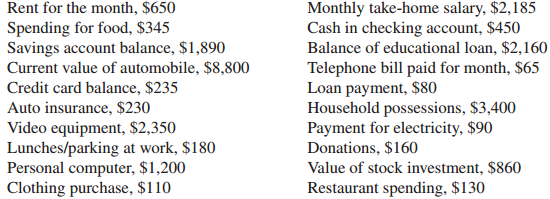### Create an Account

Home / Questions / The Fram family has liabilities of \$128000 and a net worth of \$340000 What is their debt r...

# The Fram family has liabilities of \$128000 and a net worth of \$340000 What is their debt ratio How would you assess this 2 Carl Lester has liquid assets of \$2680 and current liabilities of

The Fram family has liabilities of \$128,000 and a net worth of \$340,000. What is their debt ratio? How would you assess this?

2. Carl Lester has liquid assets of \$2,680 and current liabilities of \$2,436. What is his current ratio? What comments do you have about this financial position?

3. Use the following items to determine the total assets, total liabilities, net worth, total cash inflows, and total cash outflows.May 16 2020 View more View LessSubscribe To Get Solution